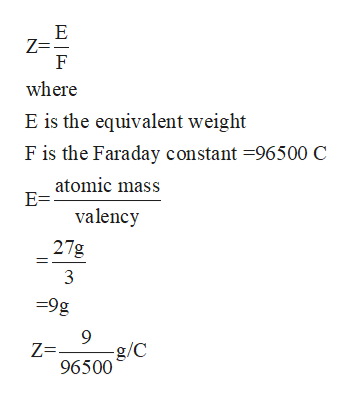# In the Hall-Heroult process, a large electric current is passed through a solution of aluminum oxide Al2O3 dissolved in molten cryolite Na3AlF6, resulting in the reduction of the Al2O3 to pure aluminum. Suppose a current of 920.A is passed through a Hall-Heroult cell for 86.0 seconds. Calculate the mass of pure aluminum produced. Be sure your answer has a unit symbol and the correct number of significant digits.

Question
267 views

In the Hall-Heroult process, a large electric current is passed through a solution of aluminum oxide

Al2O3

dissolved in molten cryolite

Na3AlF6

, resulting in the reduction of the

Al2O3

to pure aluminum.

Suppose a current of

920.A

is passed through a Hall-Heroult cell for

86.0

seconds. Calculate the mass of pure aluminum produced.

Be sure your answer has a unit symbol and the correct number of significant digits.

check_circle

Step 1

The equation of deionization for aluminum is

Step 2

The value of valency for the above equation is 3, thereforehelp_outlineImage TranscriptioncloseE Z= F where E is the equivalent weight F is the Faraday constant =96500 C atomic mass E= valency 27g =9g 9 -g/C 96500 fullscreen
Step 3

The mass discharged...

### Want to see the full answer?

See Solution

#### Want to see this answer and more?

Solutions are written by subject experts who are available 24/7. Questions are typically answered within 1 hour.*

See Solution
*Response times may vary by subject and question.
Tagged in